# 十大经典排序算法（java实现、配图解，附源码）

2022-03-04  博客园  IT界彭于晏

# 2.《数据结构与算法》中的排序算法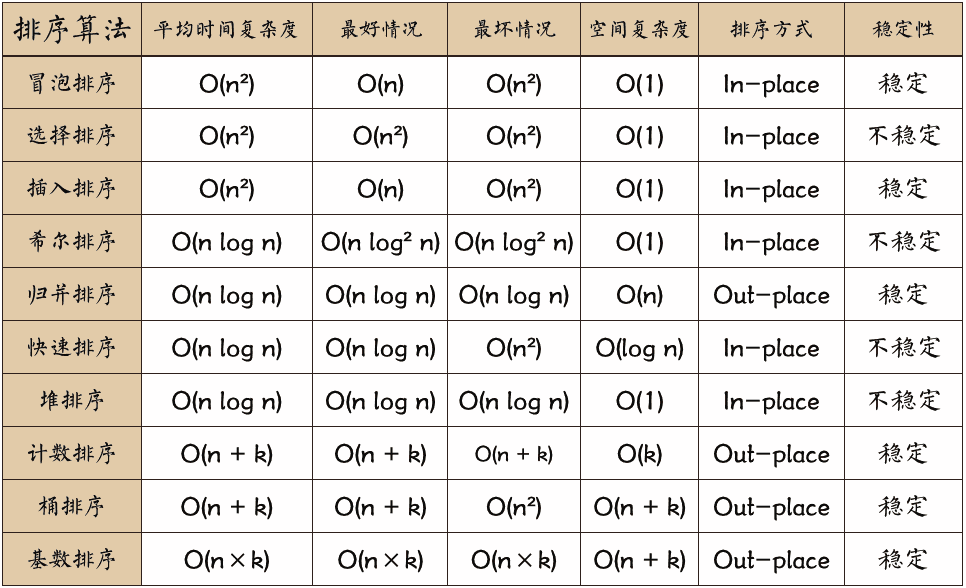# 3.算法分析

O(n1+§)) 排序，§ 是介于 0 和 1 之间的常数。 希尔排序

• n：数据规模
• k："桶"的个数
• In-place：占用常数内存，不占用额外内存
• Out-place：占用额外内存
• 稳定性：排序后 2 个相等键值的顺序和排序之前它们的顺序相同
1. 平均时间复杂度是指所有可能的输入实例均以等概率的出现情况下得到算法的运行时间
2. 最坏时间复杂度，一般讨论的时间复杂度均是最坏情况下的时间复杂度，这样做的原因是最坏情况下的时间复杂度是算法在任何输入实例上运行的界限，这就保证了算法的运行时间不会比最坏情况更长。
3. 平均时间复杂度和最坏时间复杂度是否一样，这就需要根据算法不同而不同了。

# 二、十大经典排序算法（Java开发版）

PS：案例均以数组{15，63，97，12，235，66}排序为例

# 1.1实现原理

• 比较相邻的元素。如果第一个比第二个大，就交换他们两个。
• 对每一对相邻元素做同样的工作，从开始第一对到结尾的最后一对。在这一点，最后的元素应该会是最大的数。
• 针对所有的元素重复以上的步骤，除了最后一个。
• 持续每次对越来越少的元素重复上面的步骤，直到没有任何一对数字需要比较。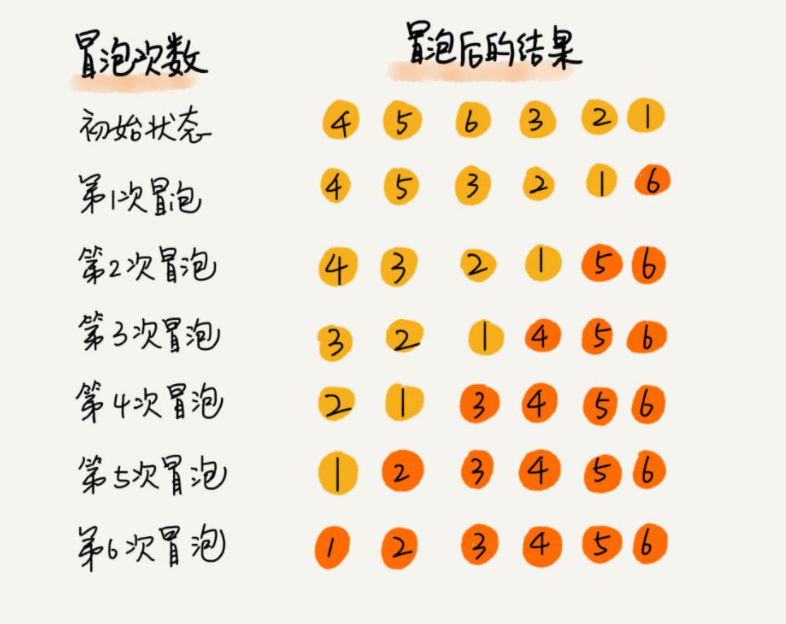# 1.2动图演示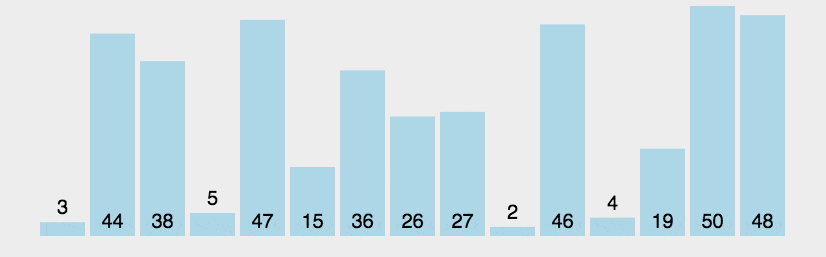# 1.3实例展示`````` 1 import java.util.Arrays;
2 public class BubbleSort {
3     public static void main(String[] args) {
4         int[] arrays =new int[]{15,63,97,12,235,66};
5         for (int i=0;i<arrays.length-1;i++){
6
7 //            控制比较次数,三者交换，实现排序
8             for(int j=0;j<arrays.length-1-i;j++){
9                 if (arrays[j] > arrays[j+1]){
10                     int temp = 0;//类似空桶
11                     temp = arrays[j]; //A桶中水倒入空桶C中
12                     arrays[j] = arrays[j+1];//把B桶的水倒入A桶中
13                     arrays[j+1] = temp;//把C桶的水倒入B桶中
14                 }
15             }
16         }
17         System.out.println(Arrays.toString(arrays));
18     }
19 }``````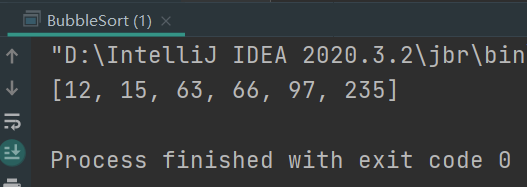# 2.1实现原理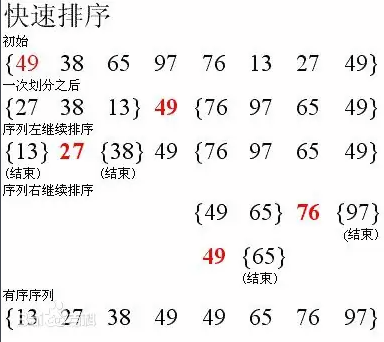# 2.2 动图演示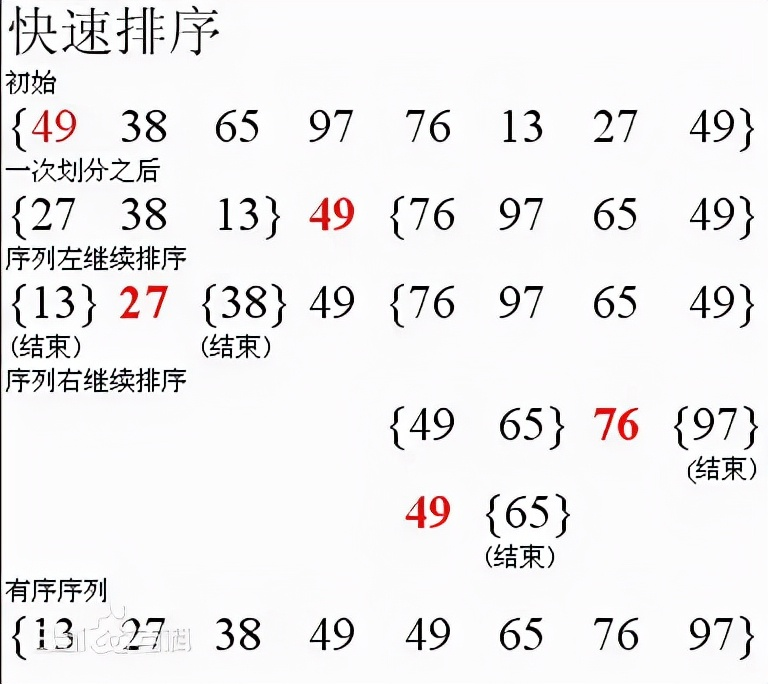# 2.3实例展示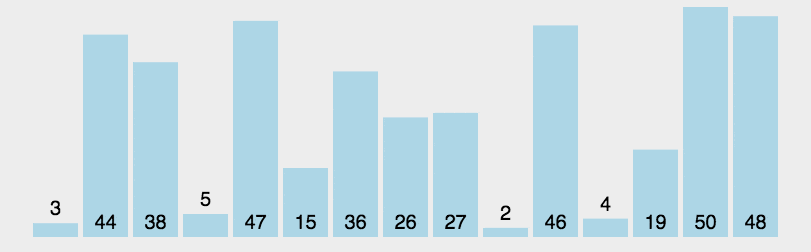`````` 1 import java.util.Arrays;
2 public class QuickSort {
3     public static void main(String[] args) {
4         int[] arrays = new int[]{15,63,97,12,235,66};
5         sort(arrays,0,arrays.length-1);
6         System.out.println(Arrays.toString(arrays));
7     }
8     public static void sort(int[] arrays,int left,int right){
9         int l  = left;
10         int r = right;
11
12         int pivot = arrays[(left+right)/2];
13         int temp = 0;
14         while (l<r){
15
16             //在左边查找小于中间值的
17             while(arrays[l]<pivot){
18                 l++;
19             }
20             //查询右边小于中间值
21             while (arrays[r]>pivot){
22                 r--;
23             }
24             if (l>=r){
25                 break;
26             }
27             temp = arrays[l];
28             arrays[l] = arrays[r];
29             arrays[r] = temp;
30
31 //            交换完数据arrays[l] = pivot
32             if (arrays[l]==pivot){
33                 r--;
34             }
35             if (arrays[r]==pivot){
36                 l++;
37             }
38             if (r==l){
39                 l++;
40                 r--;
41             }
42             if (left<r){
43                 sort(arrays,left,r);
44             }
45             if (right>l){
46                 sort(arrays,l,right);
47             }
48         }
49     }
50 }``````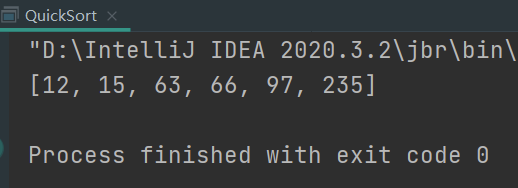# 3.1实现原理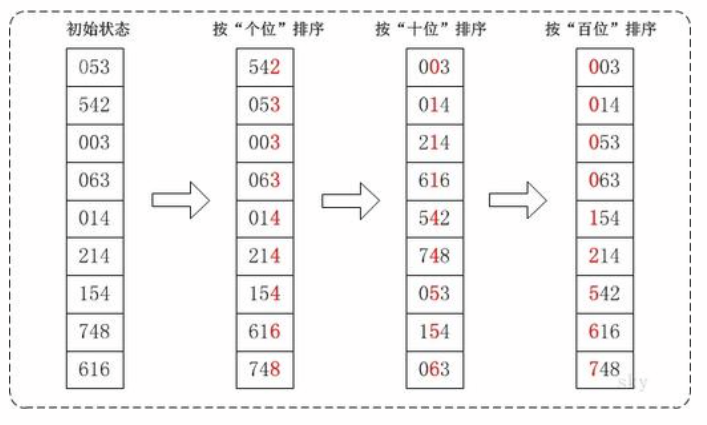# 3.2 动图演示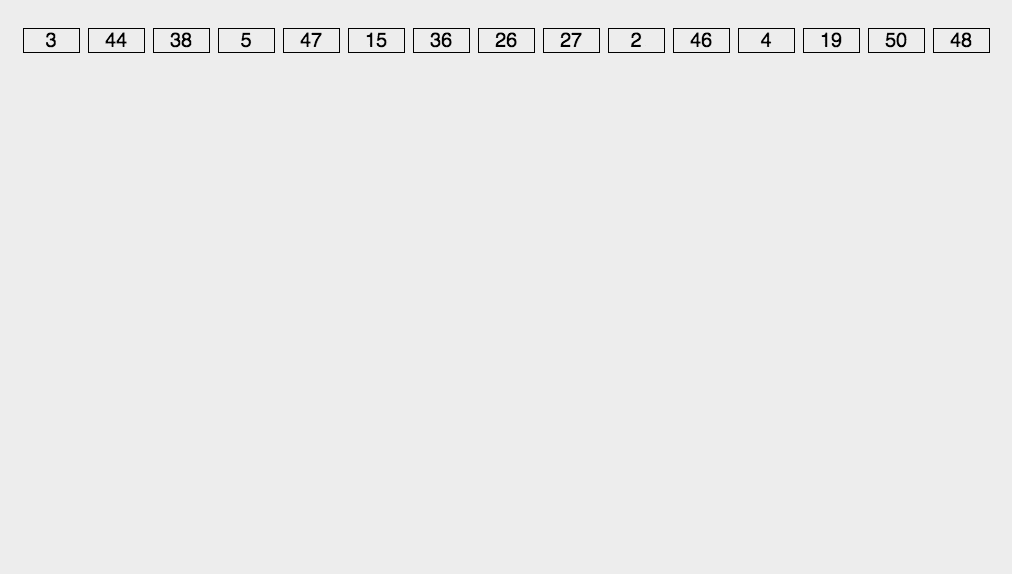# 3.3实例展示``````  1 import java.text.SimpleDateFormat;
2 import java.util.Arrays;
3 import java.util.Date;
4
5 public class BasicSort {
6
7     public static void main(String[] args) {
8         int[] arrays = new int[]{15,63,97,12,235,66};
9         SimpleDateFormat simpleDateFormat  =new SimpleDateFormat("yyyy-mm-dd HH:MM:ss:SS");
10         System.out.println("开始排序前:"+simpleDateFormat.format(new Date()));
11         sort(arrays);
12         System.out.println("排序结束:"+simpleDateFormat.format(new Date()));
13     }
14
15 //     1.获取原序列的最大位多少
16 //      @param arrays
17     public static void sort(int[] arrays){
18
19 //        获取最大位数
20         int max = 0;
21         for(int i=0;i<arrays.length;i++){
22             if (arrays[i]>max){
23                 max = arrays[i];
24             }
25         }
26
27 //        获取字符串长度，所以把int类型转字符串类型
28         int maxLength = (max+"").length();
29
30 //      定义二维数组，大小10，表示10个桶，每一个桶则是一个数组
31 //       [[],[],[],[],[]...]
32         int[][] bucket = new int[arrays.length];
33
34 //        辅助数组
35         int[] bucketElementCount = new int;
36
37 //        循环获取无序数列
38         for (int j=0;j<arrays.length;j++){
39             int locationElement = arrays[j]%10;
40
41 //            放入桶中
42             bucket[locationElement][bucketElementCount[locationElement]] = arrays[j] ;
43             bucketElementCount[locationElement]++;
44         }
45
46 //        遍历每一个桶，讲元素存放原数组中
47         int index = 0;
48         for (int k = 0;k<bucketElementCount.length;k++){
49             if (bucketElementCount[k] !=0){
50                 for (int l = 0;l<bucketElementCount[k];l++){
51                     arrays[index++] = bucket[k][l];
52                 }
53             }
54             bucketElementCount[k] = 0;
55         }
56         System.out.println(Arrays.toString(arrays));
57
58 //        第一轮针对个位数进行比较
59         for (int j = 0;j<arrays.length;j++){
60             int locationElement = arrays[j]/1%10;
61
62             bucket[locationElement][bucketElementCount[locationElement]] = arrays[j];
63             bucketElementCount[locationElement]++;
64         }
65
66 //        取出来按照桶的顺序放回原数组中
67         int indx = 0;
68         for (int k = 0;k<bucketElementCount.length;k++){
69             if (bucketElementCount[k] !=0){
70                 for (int l=0;l<bucketElementCount[k];l++){
71                     arrays[indx++] = bucket[k][l];
72                 }
73             }
74             bucketElementCount[k] = 0;
75         }
76
77 //        判断十位数
78         for (int j = 0;j<arrays.length;j++){
79             int locationElement = arrays[j]/10%10;
80
81             bucket[locationElement][bucketElementCount[locationElement]] = arrays[j];
82             bucketElementCount[locationElement]++;
83         }
84
85 //        取出来按照桶的顺序放回原数组中
86         indx = 0;
87         for (int k = 0;k<bucketElementCount.length;k++){
88             if (bucketElementCount[k] !=0){
89                 for (int l=0;l<bucketElementCount[k];l++){
90                     arrays[indx++] = bucket[k][l];
91                 }
92             }
93             bucketElementCount[k] = 0;
94         }
95
96 //        获取百位数比较
97         for (int j = 0;j<arrays.length;j++){
98             int locationElement = arrays[j]/100%10;
99
100             bucket[locationElement][bucketElementCount[locationElement]] = arrays[j];
101             bucketElementCount[locationElement]++;
102         }
103
104 //        取出来按照桶的顺序放回原数组中
105         indx = 0;
106         for (int k = 0;k<bucketElementCount.length;k++){
107             if (bucketElementCount[k] !=0){
108                 for (int l=0;l<bucketElementCount[k];l++){
109                     arrays[indx++] = bucket[k][l];
110                 }
111             }
112             bucketElementCount[k] = 0;
113         }
114         System.out.println("基数排序后的顺序:"+Arrays.toString(arrays));
115     }
116 }``````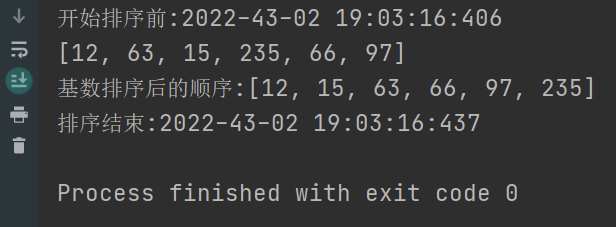# 4.1实现原理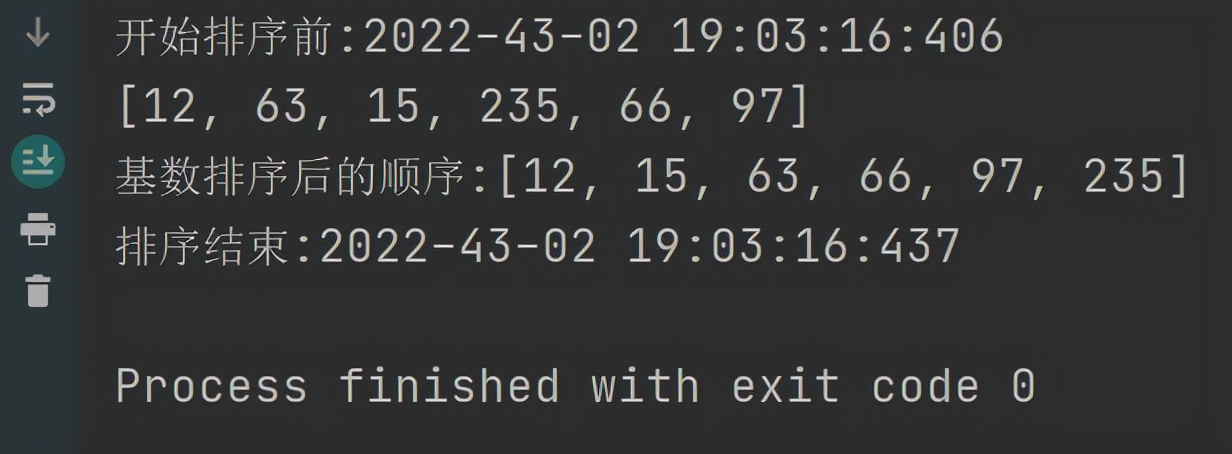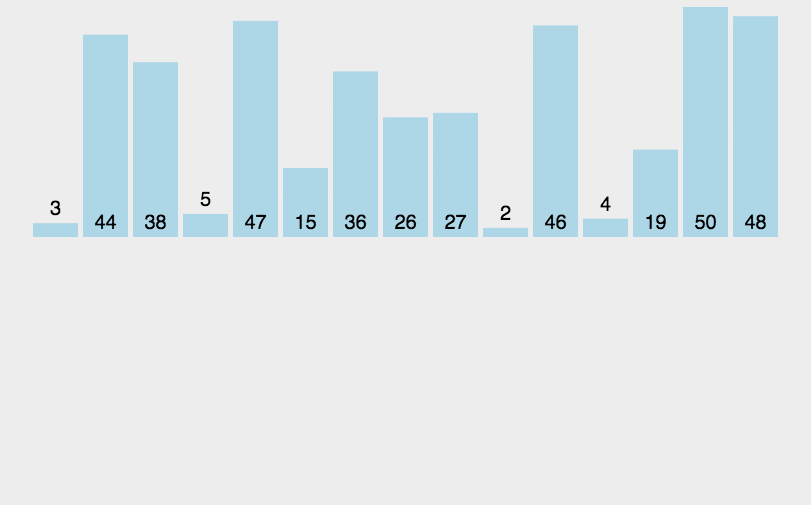# 4.3实例展示`````` 1 public class InsertSort {
2     public static void main(String[] args) {
3         int[] array = new int[]{15,63,97,12,235,66};
4         //控制拿去每一个元素
5         for(int i=1;i<array.length;i++){
6             //比较次数
7             for (int j=i;j>=1;j--){
8                 //是否小于前面的元素
9                 if (array[j]<array[j-1]){
10                     int temp = 0;
11                     temp = array[j];
12                     array[j] = array[j-1];
13                     array[j-1] = temp;
14                 }else {
15                     //continue 与 break
16                     break;
17                 }
18             }
19         }
20         System.out.println("排序后的结果："+ Arrays.toString(array));
21     }
22 }``````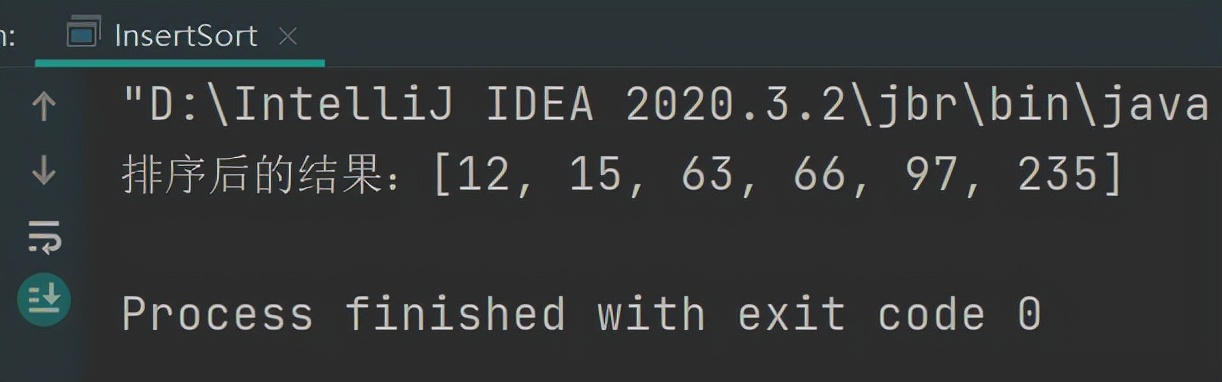# 5.1实现原理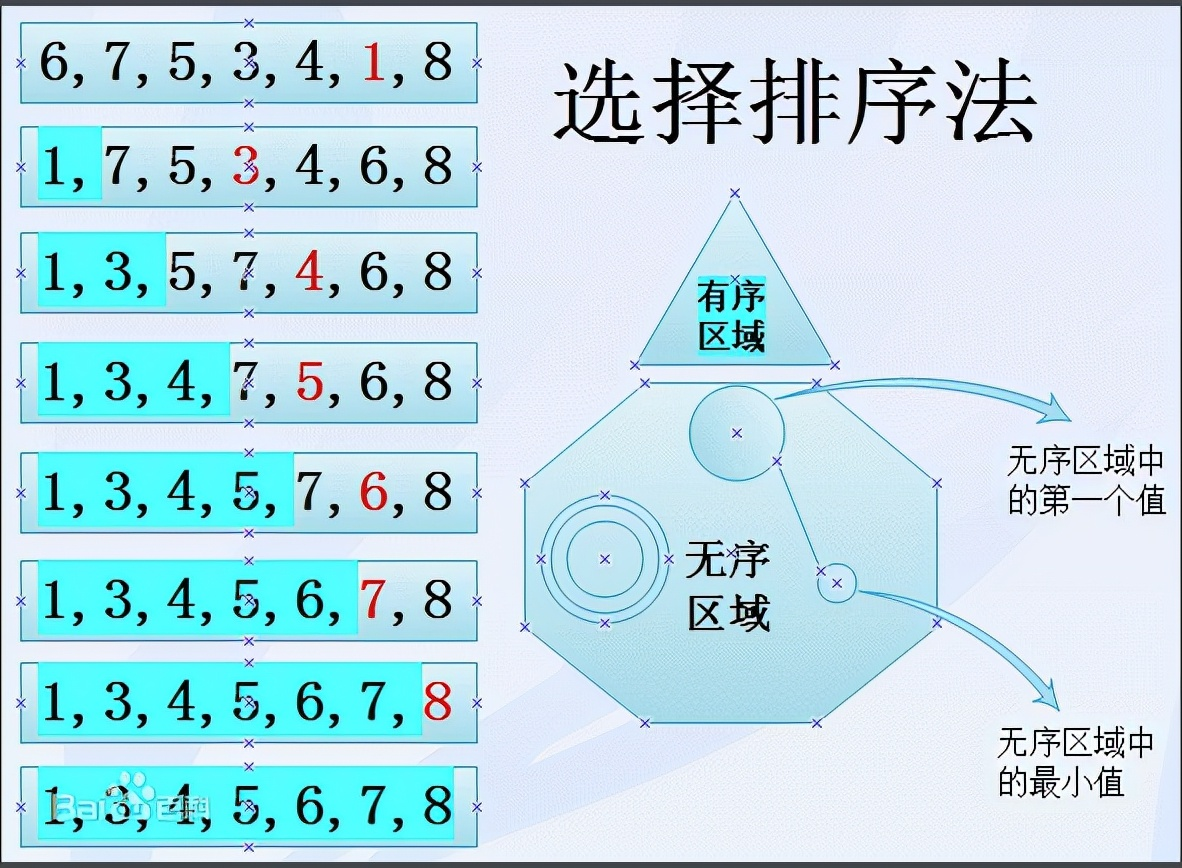# 5.2 动图演示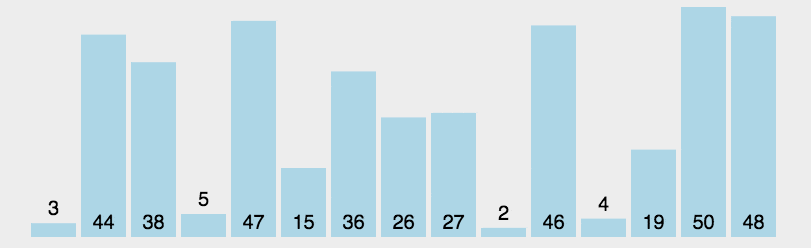# 5.3实例展示

`````` 1 import java.util.Arrays;
2 public class SelectSort {
3     public static void main(String[] args) {
4         int[] arr = new int[]{15,63,97,12,235,66};
5         for (int i=0;i<arr.length;i++){
6
7             for (int j=arr.length-1;j>i;j--){
8
9                 if (arr[j]<arr[i]){
10
11                     int temp = 0;
12                     temp = arr[j];
13                     arr[j] = arr[i];
14                     arr[i] = temp;
15                 }
16             }
17         }
18         System.out.println("选择排序后的结果:"+ Arrays.toString(arr));
19     }
20 }``````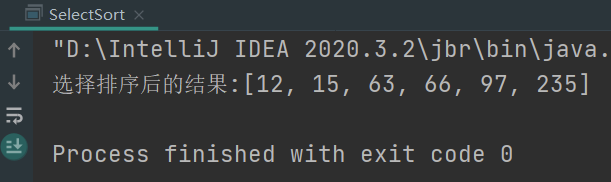# 6.1实现原理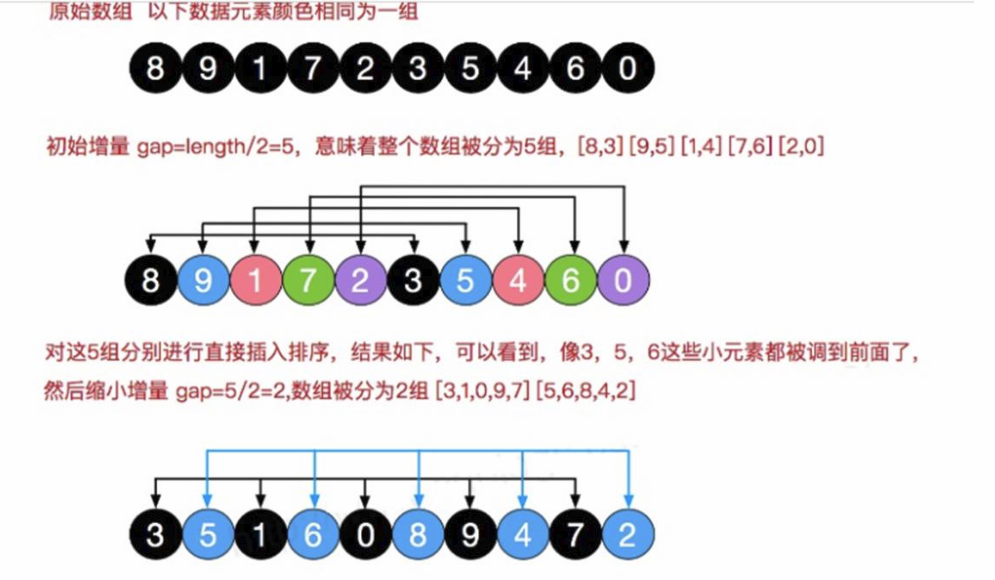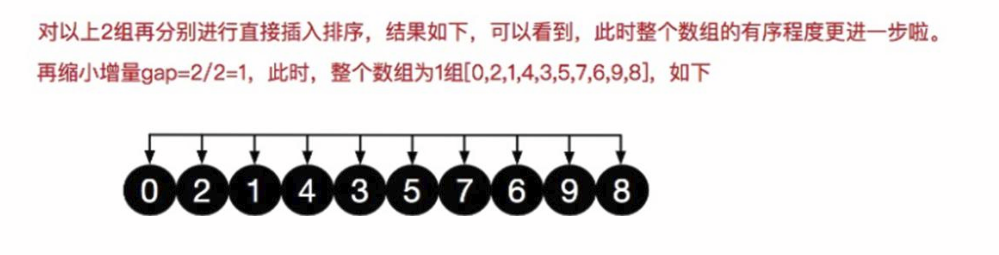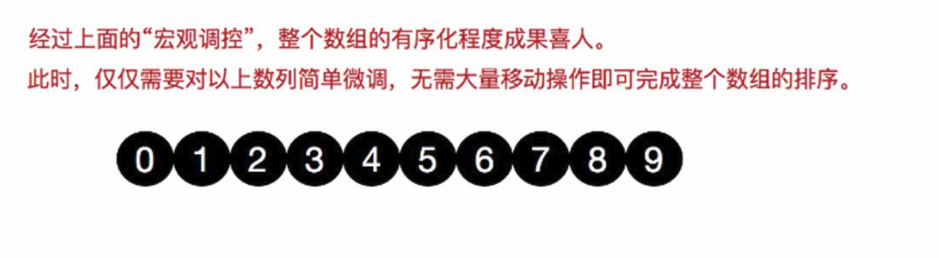# 6.2 动图演示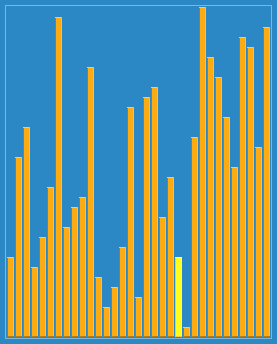# 6.3实例展示

`````` 1 import java.util.Arrays;
2 public class ShellSort {
3     public static void main(String[] args) {
4         int[] array = new int[]{15,63,97,12,235,66};
5
6 //        实现增量变化
7         for (int gap = array.length/2;gap>0;gap/=2){
8
9             for (int i=gap;i<array.length;i++){
10
11                 for (int j=i-gap;j>=0;j-=gap){
12                     if (array[j]>array[j+gap]){
13                         int temp = 0;
14                         temp = array[j];
15                         array[j] = array[j+gap];
16                         array[j+gap] = temp;
17                     }
18                 }
19             }
20         }
21         System.out.println(Arrays.toString(array));
22     }
23 }``````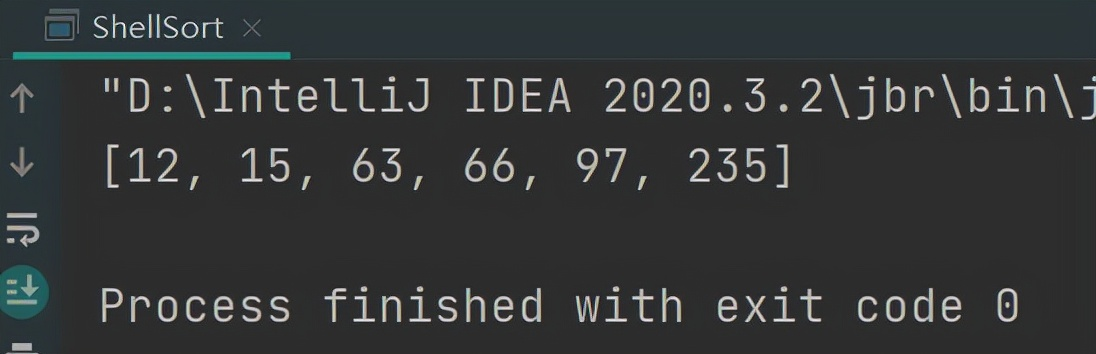# 7.1实现原理

• 第一步：申请空间，使其大小为两个已经排序序列之和，该空间用来存放合并后的序列
• 第二步：设定两个指针，最初位置分别为两个已经排序序列的起始位置
• 第三步：比较两个指针所指向的元素，选择相对小的元素放入到合并空间，并移动指针到下一位置重复步骤3直到某一指针超出序列尾将另一序列剩下的所有元素直接复制到合并序列尾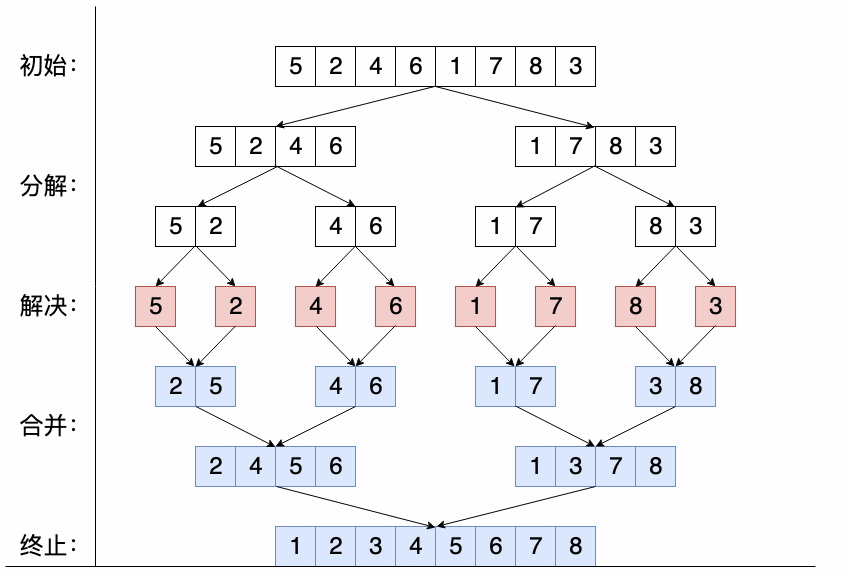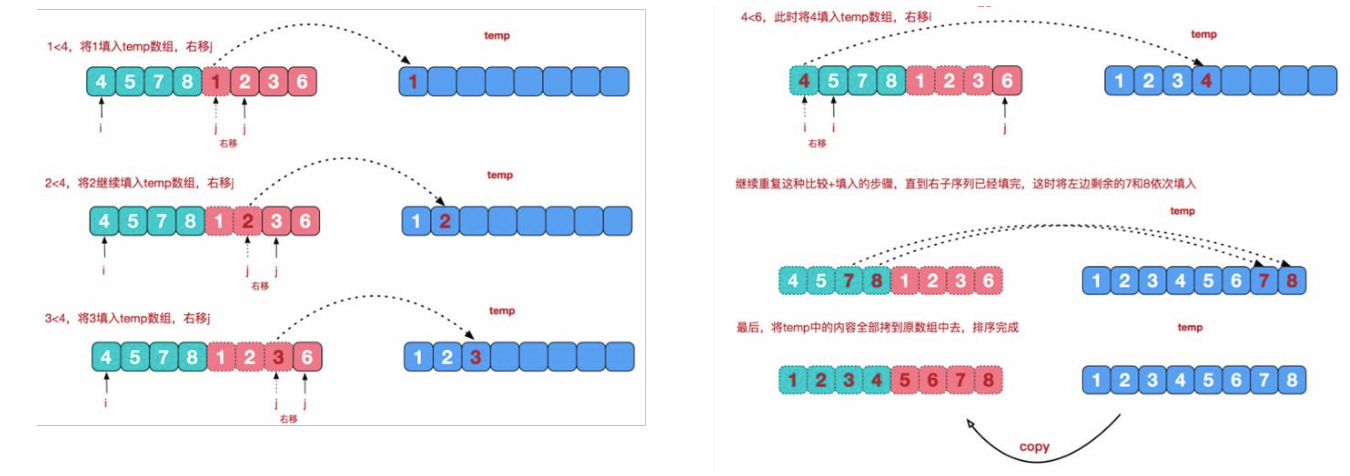# 7.2 动图演示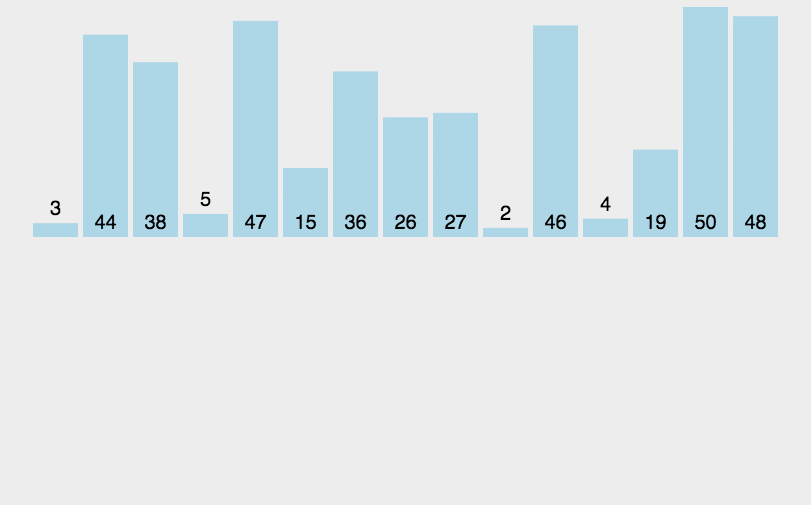# 7.3实例展示`````` 1 import java.util.Arrays;
2 public class MSort {
3     public static void main(String[] args) {
4         int[] array = new int[]{15,63,97,12,235,66};
5         //临时数组
6         int[] temp = new int[array.length];
7         sort(array,0,array.length-1,temp);
8         System.out.println(Arrays.toString(array));
9
10     }
11     public static void sort(int[] array,int left,int right,int[] temp){
12         if (left<right){
13
14 //            求出中间值
15             int mid = (left+right)/2;
16
17 //            向左边分解
18             sort(array,left,mid,temp);
19 //            向右边分解
20             sort(array,mid+1,right,temp);
21 //            合并数据
22             sum(array,left,right,mid,temp);
23         }
24     }
25     /**
26      * 合并元素
27      * @param array
28      * @param left
29      * @param right
30      * @param mid
31      * @param temp
32      */
33     public static void sum(int[] array,int left,int right,int mid,int[] temp){
34         int i = left;
35         int j = mid+1;
36
37 //        指向临时数组下标
38         int t = 0;
39
40 //        开始循环比较左右两遍数组元素比较
41         while (i<=mid && j<=right){
42
43             if (array[i]<=array[j]){
44                 temp[t] = array[i];
45                 t++;
46                 i++;
47             }else {
48                 temp[t] = array[j];
49                 t++;
50                 j++;
51             }
52         }
53
54 //        把剩余的元素直接存放在临时数组中
55         while(i<=mid){
56             temp[t] = array[i];
57             t++;
58             i++;
59         }
60         while (j<=right){
61             temp[t] = array[j];
62             t++;
63             j++;
64         }
65
66 //        临时数组中的元素拷贝至原数组中
67         int tempIndex = left;
68         int k = 0;
69         while (tempIndex<=right){
70             array[tempIndex] = temp[k];
71             k++;
72             tempIndex++;
73         }
74     }
75 }``````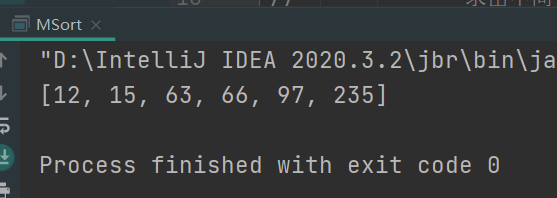# 8.1实现原理

1. 扫描整个集合S，对每一个Si∈S，找到在线性表L中小于等于Si的元素的个数T(Si)；
2. 扫描整个线性表L，对L中的每一个元素Li，将Li放在输出线性表的第T(Li)个位置上，并将T(Li)减1。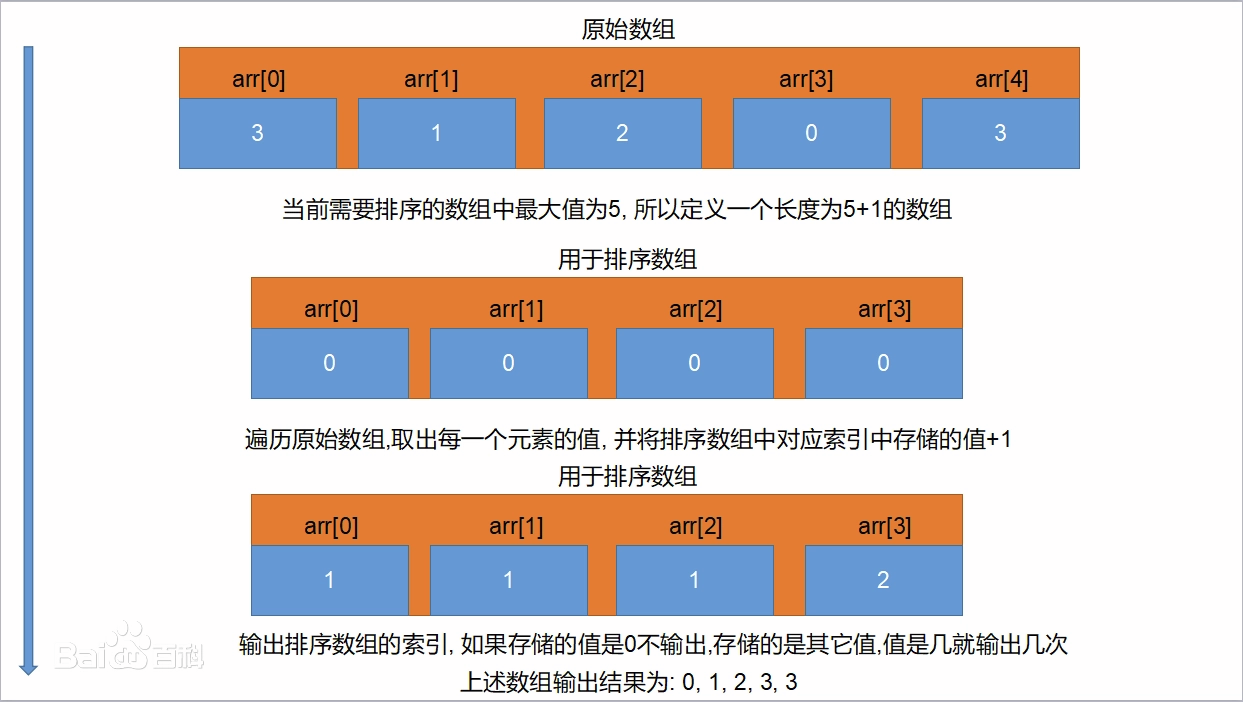# 8.2 动图演示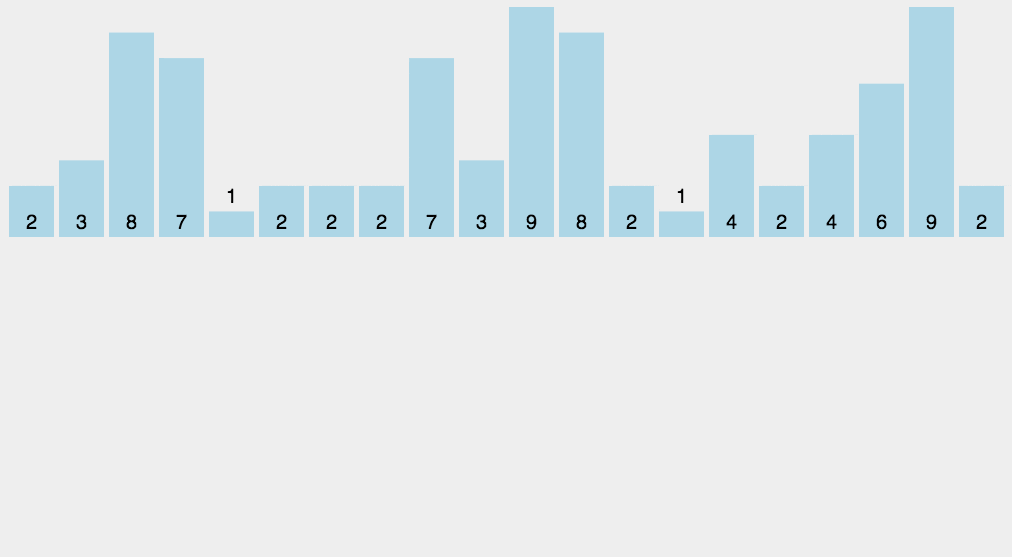# 8.3实例展示`````` 1 public class CountSort {
2     public static void main(String[]args){
3         //排序的数组
4         int a[]={15,63,97,12,235,66};
5         int b[]=countSort(a);
6         for(int i:b){
7             System.out.print( i+",");
8         }
9         System.out.println();
10     }
11     public static int[] countSort(int[]a){
12         int b[] = new int[a.length];
13         int max = a,min = a;
14         for(int i:a){
15             if(i>max){
16                 max=i;
17             }
18             if(i<min){
19                 min=i;
20             }
21         }//这里k的大小是要排序的数组中，元素大小的极值差+1
22         int k=max-min+1;
23         int c[]=new int[k];
24         for(int i=0;i<a.length;++i){
25             c[a[i]-min]+=1;//优化过的地方，减小了数组c的大小
26         }
27         for(int i=1;i<c.length;++i){
28             c[i]=c[i]+c[i-1];
29         }
30         for(int i=a.length-1;i>=0;--i){
31             b[--c[a[i]-min]]=a[i];//按存取的方式取出c的元素
32         }
33         return b;
34     }
35 }``````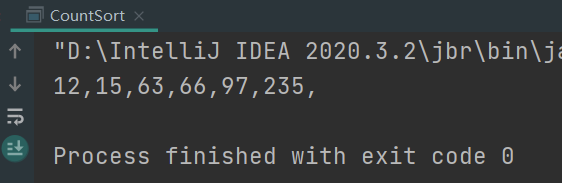# 9.1实现原理

1. 创建一个堆 H[0……n-1]；
2. 把堆首（最大值）和堆尾互换；
3. 把堆的尺寸缩小 1，并调用 shift_down(0)，目的是把新的数组顶端数据调整到相应位置；
4. 重复步骤 2，直到堆的尺寸为 1。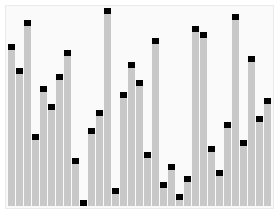# 9.2 动图演示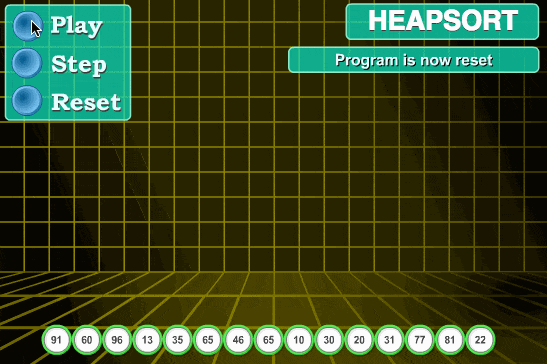# 9.3实例展示`````` 1 public static int[] heapSort(int[] array) {
2         //这里元素的索引是从0开始的,所以最后一个非叶子结点array.length/2 - 1
3         for (int i = array.length / 2 - 1; i >= 0; i--) {
4             adjustHeap(array, i, array.length);  //调整堆
5         }
6
7         // 上述逻辑，建堆结束
8         // 下面，开始排序逻辑
9         for (int j = array.length - 1; j > 0; j--) {
10             // 元素交换,作用是去掉大顶堆
11             // 把大顶堆的根元素，放到数组的最后；换句话说，就是每一次的堆调整之后，都会有一个元素到达自己的最终位置
12             swap(array, 0, j);
13             // 元素交换之后，毫无疑问，最后一个元素无需再考虑排序问题了。
14             // 接下来我们需要排序的，就是已经去掉了部分元素的堆了，这也是为什么此方法放在循环里的原因
15             // 而这里，实质上是自上而下，自左向右进行调整的
16             adjustHeap(array, 0, j);
17         }
18         return array;
19     }
20
21     /**
22     * 整个堆排序最关键的地方
23     * @param array 待组堆
24     * @param i 起始结点
25     * @param length 堆的长度
26     */
27     public static void adjustHeap(int[] array, int i, int length) {
28         // 先把当前元素取出来，因为当前元素可能要一直移动
29         int temp = array[i];
30         for (int k = 2 * i + 1; k < length; k = 2 * k + 1) {  //2*i+1为左子树i的左子树(因为i是从0开始的),2*k+1为k的左子树
31             // 让k先指向子节点中最大的节点
32             if (k + 1 < length && array[k] < array[k + 1]) {  //如果有右子树,并且右子树大于左子树
33                 k++;
34             }
35             //如果发现结点(左右子结点)大于根结点，则进行值的交换
36             if (array[k] > temp) {
37                 swap(array, i, k);
38                 // 如果子节点更换了，那么，以子节点为根的子树会受到影响,所以，循环对子节点所在的树继续进行判断
39                     i  =  k;
40                         } else {  //不用交换，直接终止循环
41                 break;
42             }
43         }
44     }
45
46     /**
47     * 交换元素
48     * @param arr
49     * @param a 元素的下标
50     * @param b 元素的下标
51     */
52     public static void swap(int[] arr, int a, int b) {
53         int temp = arr[a];
54         arr[a] = arr[b];
55         arr[b] = temp;
56     }``````# 10.1实现原理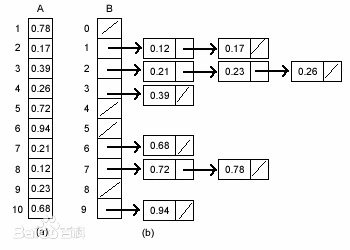# 10.2 动图演示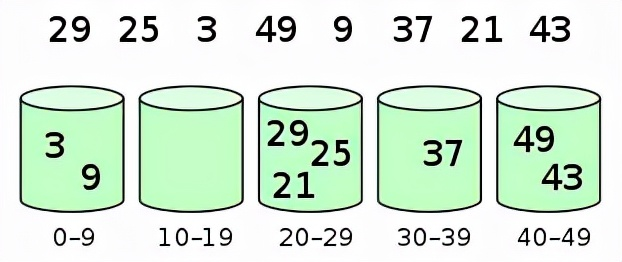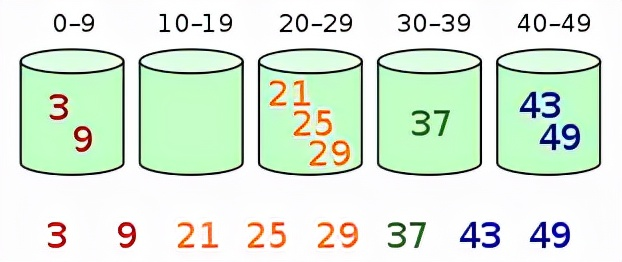`````` 1 public static void basket(int data[])//data为待排序数组
2 {
3 int n=data.length;
4 int bask[][]=new int[n];
5 int index[]=new int;
6 int max=Integer.MIN_VALUE;
7 for(int i=0;i<n;i++)
8 {
9 max=max>(Integer.toString(data[i]).length())?max:(Integer.toString(data[i]).length());
10 }
11 String str;
12 for(int i=max-1;i>=0;i--)
13 {
14 for(int j=0;j<n;j++)
15 {
16 str="";
17 if(Integer.toString(data[j]).length()<max)
18 {
19 for(int k=0;k<max-Integer.toString(data[j]).length();k++)
20 str+="0";
21 }
22 str+=Integer.toString(data[j]);
24 }
25 int pos=0;
26 for(int j=0;j<10;j++)
27 {
28 for(int k=0;k<index[j];k++)
29 {
31 }
32 }
33 for(intx=0;x<10;x++)index[x]=0;
34 }
35 }``````# 总结：

https://www.cnblogs.com/zbcxy506/p/zbcxy506_3arithmetic-01.html

▍相关推荐